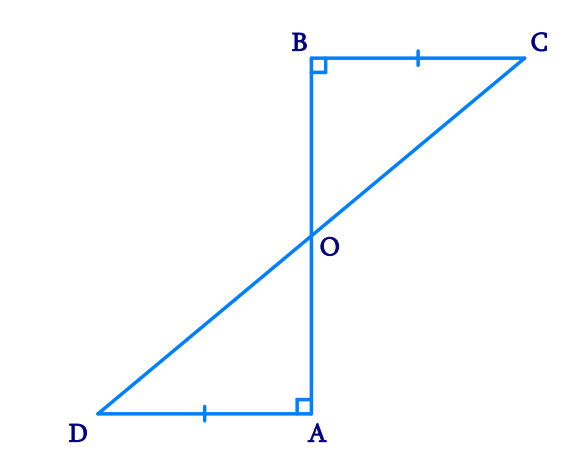In the verge of coronavirus pandemic, we are providing FREE access to our entire Online Curriculum to ensure Learning Doesn't STOP!

# Ex.7.1 Q3 Triangles Solution - NCERT Maths Class 9

Go back to  'Ex.7.1'

## Question

$$AD$$ and $$BC$$ are equal perpendiculars to a line segment $$AB$$ (See the given figure). Show that $$CD$$ bisects $$AB$$.

Video Solution
Triangles
Ex 7.1 | Question 3

## Text Solution

What is Known?

$${\text{AD}} \bot {\text{AB, BC}} \bot {\text{AB}}\;{\text{and AD}} = {\text{BC}}$$

To prove:

$$CD$$ bisects $$AB$$ or $$OA = OB$$

Reasoning:

We can show two triangles $$OBC$$ and $$OAD$$ congruent by using AAS congruency rule and then we can say corresponding parts of congruent triangles will be equal.Steps:

In $$\Delta BOC$$ and $$\Delta AOD$$,

\begin{align}\angle BOC& = \angle AOD\\\text{(Vertically opp}&\text{osite angles)}\\\\\angle CBO &= \angle DAO\text{(Each}\, 90^ {\circ})\\BC &= AD \text{(Given)}\\\therefore \Delta BOC &\cong \Delta AOD\\ \text{(AAS} \,& \text{congruence rule)}\\\\\therefore BO &= AO \text{(By CPCT)}\end{align}

Hence $$CD$$ bisects $$AB$$.

Video Solution
Triangles
Ex 7.1 | Question 3

Learn from the best math teachers and top your exams

• Live one on one classroom and doubt clearing
• Practice worksheets in and after class for conceptual clarity
• Personalized curriculum to keep up with school